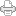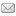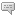## Algebra of Tetraktys, Geometry of Larson's Cube & the Wheel of Motion

Posted on Saturday, December 10, 2011 at 08:33AM

When I first showed the LRC’s RST-based “Wheel of Motion” to our new LRC associate, Larry, the mathematician, he said, “Well that’s nice, but how come you don’t make it 3D?”

I’ve always hated that question, because in a 3D universe, the periodic table of elements ought to be 3D, but the wheel of motion, like the periodic table, is only a 2D representation of the periods of its elements. It has always seemed to me that showing the true periodic nature of the elements in the wheel format, which eliminates the confusing gaps in the table format, was accomplishment enough, especially since the wheel format clearly shows the true magnitudes of the RST-based 4n2 numbers, rather than quantum mechanics (QM)-based 2n2 half-period numbers of the table.

Well, it turns out that several years ago, studying the numbers of the tetraktys, we discovered how they are actually the algebraic equivalent of the geometry of Larson’s Cube. Since then, we’ve been trying to understand the SUDR and TUDR, and their combinations as preons, which we call S|T units that combine to form the so-called “fundamental particles” of our preon version of the standard model, and connect them to the wheel. These entities combine to form the seqence of elements in the wheel, following a 4+16+36+64 = 120 pattern of mathematical “slots” for the 118 elements plus the proton and the neutron.

It’s not been easy, but we have made some progress, with many starts and stops along the way. One important connection links the progression of the LC (and thus the tetraktys) with the wheel of motion. We’ve found that we can encode the universal space/time expansion in terms of the expanding LC, which can be written as the expanding 3D level of the tetraktys

TEn = ne0 (+) 2(ne0)1 (+) 4(ne0)2  (+)  8(ne0)3

where TEn is the expanded LC equivalent of the tetraktys, n is the unit variable of its time expansion, and e0 is the scalar time unit (i.e. e0 = 1, the point, but it also represents one line, one square and one cube, after one unit of time expansion), corresponding to one edge of the LC (measured from the interior center point of the LC to the face center, lying along any one of the three axes of the stack of 8 one-unit cubes, making up the LC.)

So, in 1 unit of time, the value of TEn is

TE1 = 1*10 (+) 2*11 (+) 4*12  (+)  8*13   = 1 (+) 2 (+) 4 (+) 8

The (+) operator indicates the joining together of the four components of the LC, the respective values of the scalar point unit, the linear line unit, the square area unit, and the cubic volume unit of the LC, into one, unified, entity, isomorphic to the 3D line of the tetraktys (20, 21, 22, 23).

This is similar to the concept of joining of the scalar, vector, bivector and trivector into a multivector in Hestenes’ Geometric Algebra. Only, in our case, we join the 0D scalar and the three pseudoscalars of the tetraktys into the LC.

Hence, at t=2,

TE2 = 2e0 (+) 2(2e0)1 (+) 4(2e0)2  (+)  8(2e0)3   = 2 + 4 + 16 + 64

Similarly,

TE3 = 3 + 6 + 36 + 216,

and

TE4 = 4 + 8 + 64 + 512.

Now, clearly, any of the six 2x2 = 4 face units of the 2x2x2 = 8 stack of cube units of TE1 corresponds to the four slots of the innermost wheel of the Wheel of Motion, where the RST-based period is 4n2 = 4, when n = 1 (The four slots for the Proton, Neutron, Protium, Deuterium (H).)

Similarly, any one of the six 4x4 = 16 face units of the 4x4x4 = 64 stack of cubic units of TE2 corresponds to the sixteen slots of the second, outer wheel, when 4n2 = 16, n = 2.

The third wheel corresponds to one of the six 6x6 = 36 face units of the 6x6x6 = 216 cubic units of TE3, where the RST-based period is 4n2 = 36, n = 3, and the fourth wheel corresponds to one of the six 8x8 = 64 face units of the 8x8x8 = 512 stack of cubic units of TE4, where the RST-based period is 4n2 = 64, n = 4.

Of course, the question is, how do we know that this is anything other than a mathematical coincidence? Where is the physical connection to these numbers? Well, the answer is that we are still trying to clarify that connection, but it’s certainly interesting to note that the ratios of the associated balls of the LC follow this same numerical pattern that we see in the expanded right lines.

That is to say, the ratios of the respective radii, surfaces and volumes of the two balls associated with TE1, TE2, TE3 and TE4 (the outer ball and its inverse), follow the same numerical pattern as do the edges, lines, squares and cubes in the expanding LC, because, as it turns out, the ratio of the radius of an initial volume to that of the nth volume of equal magnitude added to it, is equal to the cube root of n. Everything follows from there.

If we take the outer ball of the LC, which geometry and algebra agree must have a radius equal to the square root of 3, and its inverse ball, which must have a radius equal to the inverse of the square root of 3, and follow their expansion as the LC expands, we find that it takes the sum of the volume of 8 balls in each case to expand the ratio of the radius of the initial ball to the radius of the nth ball from 1 to 2, (81/3 = 2).

It then takes 64 of these volumes to expand the ratio of the two radii to 4, (641/3 = 4). It takes 216 volumes to expand the ratio to 6, (2161/3 = 6), and it takes 512 volumes to expand the ratio to 8, (5121/3 = 8).

Thus, we see that there is not only a 0D, 1D, 2D and 3D mathematical (discrete unit) connection between the algebra of the tetraktys, the geometry of the LC and the Wheel of Motion, but there is also found a corresponding physical (i.e. continuous unit) connection between them.

Moreover, we see that it is the area aspect of a given volume that yields the 4n2 periods of the wheel, which means that the associated sums of the 3D volumes are actually incremented to form the 2D elemental slots in each period; That is, summing the volumes leads to the RST’s 4n2 relation, because the six faces of each expanded stack of one-unit cubes are degenerate. Thus, only one need be selected to select associated two-unit volumes. Consequently, since the smallest face possible has a magnitude of four squares, when the successive expanded LCs are divided by 4, we get the required relation:

4/4 = 12; 16/4 = 22; 36/4 = 32; 64/4 = 42.

And because this is so, we can just as easily represent the periods in the wheel as a factor of cubes:

8n3 = 8, n=1; 8n3 = 64, n= 2, 8n3 = 216, n=3; 8n3 = 512, n=4,

which is just the cubic progression of the LC.

So, to answer Larry, from a graphics perspective, it’s just much easier to draw a 2D wheel of motion than it would be to draw a 3D version. However, from a mathematical perspective, the 2D aspect of the wheel cannot be separated from its 3D aspect, because, in reality, they are simply two aspects of the same thing.

The bottom line is, even though the Wheel of Motion is not drawn in 3D, it represents a 3D volume sequence nevertheless.

Now, we need to find the way to build the expanded LCs (TE1, TE2, TE3, TE4), using the S|T units that serve as the preons to our standard model of particles. Some ideas on that next.View Printer Friendly VersionEmail Article to Friend

### Reader Comments

There are no comments for this journal entry. To create a new comment, use the form below.

###Post a New Comment

Enter your information below to add a new comment.

My response is on my own website »
Author Email (optional):
Author URL (optional):
Post:

Some HTML allowed: <a href="" title=""> <abbr title=""> <acronym title=""> <b> <blockquote cite=""> <code> <em> <i> <strike> <strong>